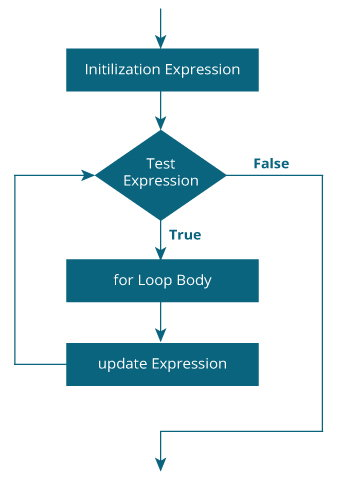# What is a for loop in c

In programming, loops are used to repeat a block of code until a specified condition is met.
C programming has three types of loops:
1. for loop
2. while loop
3. do...while loop
We will learn about `for` loop in this tutorial. In the next tutorial, we will learn about `while` and `do...while` loop.

## for Loop

The syntax of the `for` loop is:
```
`for (initializationStatement; testExpression; updateStatement)`
`{`
`    // statements inside the body of loop`
`}`

```

### How for loop works?

• The initialization statement is executed only once.
• Then, the test expression is evaluated. If the test expression is evaluated to false, the `for` loop is terminated.
• However, if the test expression is evaluated to true, statements inside the body of `for` loop are executed, and the update expression is updated.
• Again the test expression is evaluated.
This process goes on until the test expression is false. When the test expression is false, the loop terminates.
To learn more about test expression (when the test expression is evaluated to true and false), check out relational and logical operators.

### for loop Flowchart### Example 1: for loop

```
` # Print numbers from 1 to 10`
`#include <stdio.h>`

`int main() {`
`  int i;`

`  for (i = 1; i < 11; ++i)`
`  {`
`    printf("%d ", i);`
`  }`
`  return 0;`
`}`

```
Output
`1 2 3 4 5 6 7 8 9 10`
1. i is initialized to 1.
2. The test expression `i < 11` is evaluated. Since 1 less than 11 is true, the body of `for` loop is executed. This will print the 1 (value of i) on the screen.
3. The update statement `++i` is executed. Now, the value of i will be 2. Again, the test expression is evaluated to true, and the body of for loop is executed. This will print 2 (value of i) on the screen.
4. Again, the update statement `++i` is executed and the test expression `i < 11` is evaluated. This process goes on until i becomes 11.
5. When i becomes 11, i < 11 will be false, and the `for` loop terminates.

### Example 2: for loop

```
`// Program to calculate the sum of first n natural numbers`
`// Positive integers 1,2,3...n are known as natural numbers`

`#include <stdio.h>`
`int main()`
`{`
`    int num, count, sum = 0;`

`    printf("Enter a positive integer: ");`
`    scanf("%d", &num);`

`    // for loop terminates when num is less than count`
`    for(count = 1; count <= num; ++count)`
`    {`
`        sum += count;`
`    }`

`    printf("Sum = %d", sum);`

`    return 0;`
`}`

```